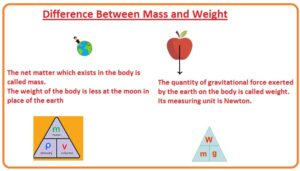comparisonHello fellows, I hope you all are doing great. In today’s tutorial, we will have a look at Difference Between Mass and Weight. There are 2 terms commonly used in physics first one is the mass and second one weight. Both terms are considered the same to each other and usually used. But in real fact, both of these are different parameters. . In today’s post, we will have a detailed look at different differences among these two parameters. So let’s get started with Difference Between Mass and Weight.

#### Difference Between Mass and Weight

Mass:

• The net matter which exists in the body is called mass.
• The weight of the body is less at the moon in place of the earth
• Mass is property used to measure the strength of the force
• Mass is a feature of an object and it also defines the resistance offed by the body
• It also explains the gravitational force at the body
• Its existence does not depend on the location.
• It is base on type quantity.
• Its value does not vary at different positions.
• It measured in grams and kilograms.
• Slug is an imperial unit of mass
• It denoted as m’.
• Mass is an inertial feature of the object
• Mass is base quantity
• Mass=volume x density
• Mass can not be zero
• can be measured through level balance
• It is a scalar quantity.
• Its numerical value can not be zero.
• Mass helps to finds acceleration. F=ma
• It can be calculated with the normal balance.• Its mathematical relation is m = w/g.
• The resistance of the body that is accelerated through force is called inertial mass.
• Gravitiaonl field is determined by the active gravitational mass
• The force applying to an object is measured by passive gravitational mass

Weight:

• The quantity of gravitational force exerted by the earth on the body is called weight.
• Its measuring unit is Newton.
• It is derived quantity
• Has different value due to different value of gravity
• It denoted as W’.
• It is a vector. In free fall weight is zero.
• All terrestrial object is considered as weightless
• Its value changes with the change in gravity.
• It calculated with the spring balance.
• Its mathematical relation is w=mg.
• the feature of the body that defines the heaviness of the body its example is the buoyancy effect
• It is a vector quantity.
• Its values contrary to mass can zero where gravity does not exist.
• It depends on the location of the body.

That is a detailed post about the difference between mass and weight if you have any query ask in the comments. Thanks for reading. Have a good day.